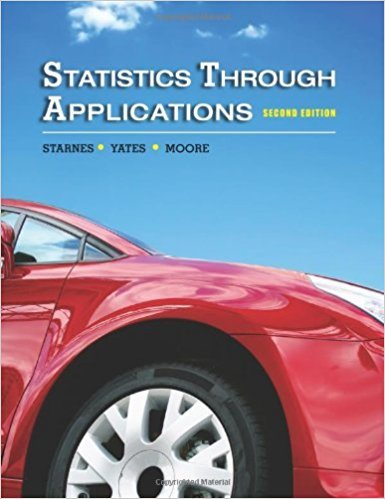×
Get Full Access to Statistics Through Applications - 2 Edition - Chapter 9 - Problem 9.67
Get Full Access to Statistics Through Applications - 2 Edition - Chapter 9 - Problem 9.67

×

# Online gaming, I A random sample of 1100 teenagers (agedISBN: 9781429219747 133

## Solution for problem 9.67 Chapter 9

Statistics Through Applications | 2nd Edition

• Textbook Solutions
• 2901 Step-by-step solutions solved by professors and subject experts
• Get 24/7 help from StudySoup virtual teaching assistantsStatistics Through Applications | 2nd Edition

4 5 1 336 Reviews
27
4
Problem 9.67

Online gaming, I A random sample of 1100 teenagers (aged 12 to 17) were asked whether they played games online; 775 said that they did. (a) Construct and interpret a 99% confi dence interval for the population proportion p. (b) Suppose that the results of the survey were used to construct separate 99% confi dence intervals for boys and girls. Would the margins of error for those two confi dence intervals be the same as, larger than, or smaller than the margin of error for the interval you constructed in (a)? Justify your answer. (c) In the context of this exercise, describe a real-world issue that isnt included in the margin of error.

Step-by-Step Solution:
Step 1 of 3

Chemistry Notes Week 13 NOTE: May 1st- Chemistry ﬁnal review session An oxidation-reduction (or redox) reaction is a chemical reaction in which electrons are transferred from one reactant to another A. Oxidation is the loss of electrons B. Reduction is the gain of electrons 2+ C. Reducing agents (Zn loses two electrons and is oxidized to Zn ) 2+ D. Oxidizing agent (Cu gains two electrons and is reduced to Cu) E. A redox reaction is the sum of an oxidation half-reaction and a reception half- reaction The oxidation number is the charge an atom if electron were transferred completely A. H2(g) + F2(g) —>

Step 2 of 3

Step 3 of 3

##### ISBN: 9781429219747

The full step-by-step solution to problem: 9.67 from chapter: 9 was answered by , our top Statistics solution expert on 11/10/17, 06:04PM. This textbook survival guide was created for the textbook: Statistics Through Applications, edition: 2. This full solution covers the following key subjects: confi, Error, dence, margin, online. This expansive textbook survival guide covers 10 chapters, and 710 solutions. Statistics Through Applications was written by and is associated to the ISBN: 9781429219747. The answer to “Online gaming, I A random sample of 1100 teenagers (aged 12 to 17) were asked whether they played games online; 775 said that they did. (a) Construct and interpret a 99% confi dence interval for the population proportion p. (b) Suppose that the results of the survey were used to construct separate 99% confi dence intervals for boys and girls. Would the margins of error for those two confi dence intervals be the same as, larger than, or smaller than the margin of error for the interval you constructed in (a)? Justify your answer. (c) In the context of this exercise, describe a real-world issue that isnt included in the margin of error.” is broken down into a number of easy to follow steps, and 113 words. Since the solution to 9.67 from 9 chapter was answered, more than 245 students have viewed the full step-by-step answer.

Unlock Textbook Solution